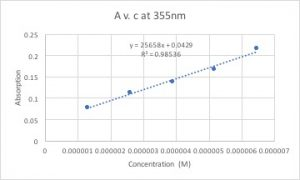# Environmentally-Friendly Energy

My name is Eric Park and I am a second-year student studying in Emory College. I am undecided, but am highly considering applying to medical school.

My Lab and Research

I am working in Dr. Dyer’s lab, specifically with my graduate mentor Greg. Greg is a third-year student studying inorganic/physical chemistry. Our research is on understanding the mechanism of hydrogen bonds in metalloenzymes—enzymes that contain a metal ion—and produce “mimic” enzymes to utilize the bond energies as an environmentally-friendly and cost efficient energy source. We also focus on the 2H+ + 2e- –> H2 reaction and study how nature efficiently carries out this reaction.

First Lab

I went in the lab for the first time on September 30th, 2017 and Greg taught me how to analyze absorption rates using a UV-Vis spectrometer. Absorption rate is the percentage of light a solution absorbs as a specific wavelength of light is shined through it. Using the equation **A= E*c*l (A= absorption, E= extinction coefficient, c= concentration, l= path length) and the absorption rate that was collected from the UV-Vis spectrometer, we can calculate the extinction coefficient—a specific absorption rate value unique for every substance. In this particular experiment, I used a diluted myoglobin solution as the subject of study.

**As concentration of the solution increases, the less light is able to shine through. This is self-explanatory by using every day examples. The more nestle chocolate milk powder you put in water, the darker the solution gets and the lesser amount of light is able to pass through. For the path length, light is less able to pass through a full tank of dark chocolate milk than a small glass of chocolate milk.

Second Lab

On October 3rd, 2017 I repeated the same experiment but with dithyanide mixed to the myoglobin solution. Dithyanide has two electrons that can reduce other molecules, therefore it can reduce two myoglobin molecules. This reduction of myoglobin changes the conformation of the ligands– anything attached to the myoglobin– and puts the electrons in a lower energy state. Since a lower energy state is equivalent to a higher wavelength, the absorption peak appeared at a higher wavelength (I will add figures later when they become available). In addition, we can calculate the extinction coefficient by graphing  A v. c and calculating the slope of the trend line. Here is a sample graph where I graphed A v. c for the dithyanide + myglobin solution:On the y-axis is the absorption rate and on the x-axis is the concentration of the solution. It is evident in the graph that the concentration and the absorption rate are proportional to each other. The slope of the trend line would be the value of the extinction coefficient because if you manipulate the equation A=E*c*l to E= A/(c*l) you can see that the absorption rate divided by the concentration gives the value of the extinction coefficient (the path length is 1 we can disregard it).

What I plan to do…

For the next couple months, I plan on learning more lab techniques and reading journals. I do not think I will actually get involved in Greg’s research until late spring to early summer. Until then, I plan on learning all the lab techniques that are required to assist Greg in his research.

## 2 Replies to “Environmentally-Friendly Energy”

1.Michael Francis Thees says:

Not a bad post, I certainly enjoyed it. A few small comments though, while you were very clear in defining your variables at no point do you describe how they are related. Also more cartoons would help, especially when you talk about energy levels for the electron in that molecule. My thought, when I first read it, was that being more tightly bound would increase the adsorption energy required (meaning a decrease in the wavelength).

Finally, you should work on your graph presentation a bit, that graph is generally hard to read.

2.Tyler Tri-Thanh Pham says:

Hi Eric! I really like reading your post. The oranization and layout of your post was really easy to follow and understand. What if you could put a background section? This would have defintely helped as some of the information you mentioned in the post was a little bit foregin to me. Overall, it was an excellent post!